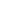Phone:954-442-1066 (09:00 AM - 5:00 PM, EST)# Calculating the Volume of a Pool in Gallons

## Home -> Help -> Pool Volume Calculations

### Calculating the volume of a Rectangular swimming Pool

For Slope Pools, Multiply the results by 0.85### Calculating the volume of a Oval Flat Bottom Swimming Pool

For Slope Pools, Multiply the results by 0.85### Calculating the volume of a Round Flat Bottom Swimming Pool

For Slope Pools, Multiply the results by 0.85